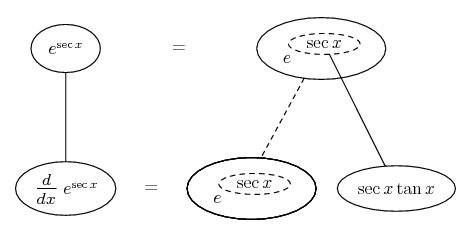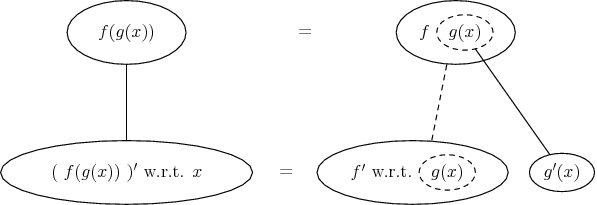# Thread: differentiation problem

1. ## differentiation problem

Is this right for the differential of e^secx

secx e^secx?

2.Originally Posted by offahengaway and chipsIs this right for the differential of e^secx

secx e^secx?
No, it should be:

$\displaystyle e^{\sec(x)}\frac{d}{dx}\sec(x)$

I will leave you to finish it.

CB

3. would that be e^sec(x).sec(x).tan(x)?

4. Yup. (As long as you mean $\displaystyle e^{\sec x} \sec x \tan x$ and not e raised to all of the rest.)Straight continuous lines differentiate downwards with respect to x, the straight dashed line with respect to the dashed balloon expression. So the forking network on the right satisfies the chain rule - the general pattern being...Don't integrate - balloontegrate!

Balloon Calculus Forum

### Differenciate (secx -tanx)/(secx tanx) with respect to x

Click on a term to search for related topics.

#### Search Tags

differentiation, problem